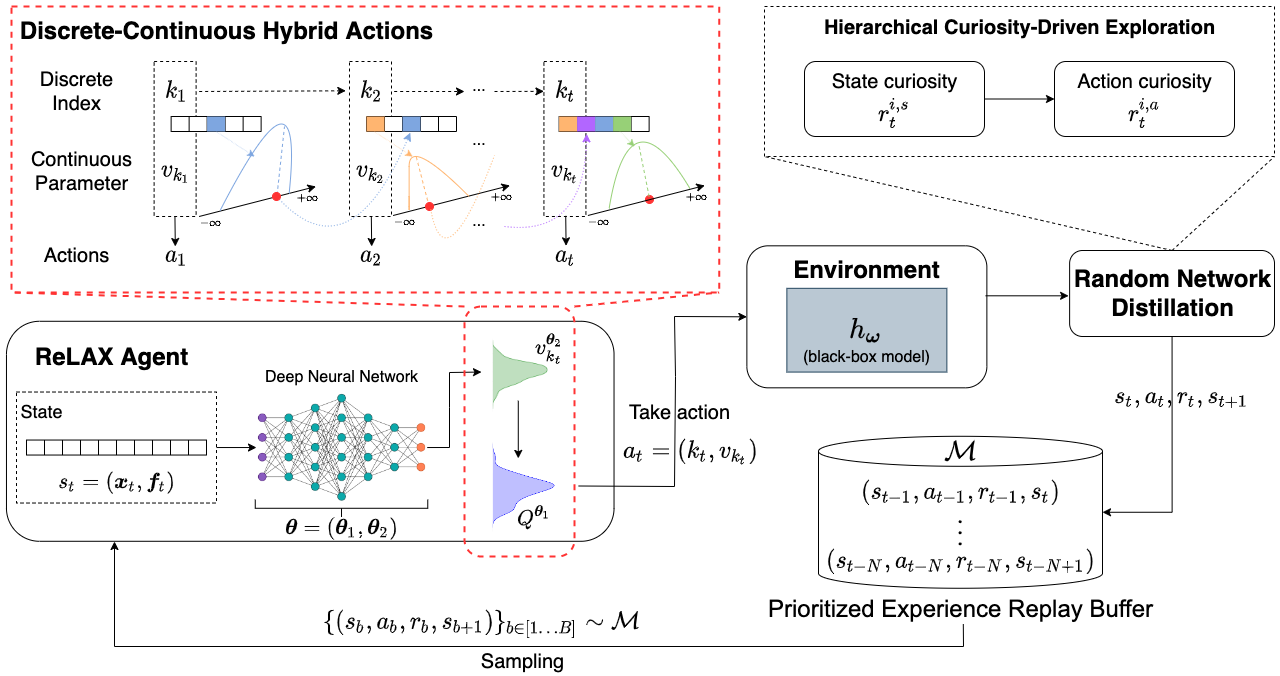ReLAX

# ReLAX

ReLAX is the first counterfactual explanation method for any black-box machine learning model, based on reinforcement learning (RL). In a nutshell, it formulates the problem of generating counterfactual examples as a Markov Decision Process (MDP) with a discrete-continuous hybrid action space. This resembles the behavior of an RL agent that, given any input instance $\boldsymbol{x}$, produces its corresponding optimal counterfactual example $\tilde{\boldsymbol{x}}^*$ by repeatedly picking one of the features to change (i.e., a discrete action) and applying a slight perturbation to it (i.e., a continuous action). More information on this work can be found at this link.